# Code with correction of deletions and insertions

(diff) ← Older revision | Latest revision (diff) | Newer revision → (diff)

code for correction of deletions and insertions

A code intended for the correction of errors of two types encountered in the transmission of information. By a deletion of a letter in a word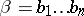of lengthover some alphabetone means a transformation ofinto a word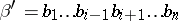of length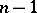,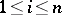. The numbersof words obtainable fromby deletion ofletters satisfies the following estimates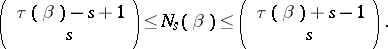Hereis the number of series of(by a series of a wordone means a word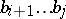,, such that 1)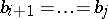; 2) if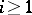, then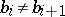; and 3) if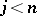, then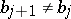). In particular,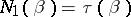. By an insertion of a letter in a wordone means a transformation ofinto a word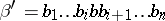of length, where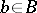and. The number of words obtainable from an arbitrary wordof lengthby the insertion ofletters of an alphabetis equal to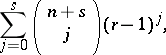whereis the number of letters in. A setof words over the alphabetis called a code for correction ofdeletions (insertions, deletions or insertions) if no word incan be obtained from two distinct words ofas the result ofor fewer deletions (insertions, deletions or insertions) of letters in each of them. The function defined on the pairs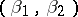of words inand equal to the minimum number of deletions and insertions of letters convertinginto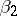is a metric. A setof words over the alphabetis a code for correction ofdeletions (insertions, deletions or insertions) if and only if the distance between any two distinct words ofis greater than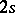, so that the above three definitions of a code are equivalent. An example of a code for correction of one deletion or one insertion is the set of words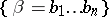of lengthover the alphabet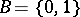for which(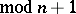). The number of words in this code is equal to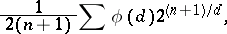where the sum is taken over all odd divisorsofand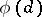is the Euler function; it is asymptotically maximal as.

How to Cite This Entry:
Code with correction of deletions and insertions. Encyclopedia of Mathematics. URL: http://encyclopediaofmath.org/index.php?title=Code_with_correction_of_deletions_and_insertions&oldid=11397
This article was adapted from an original article by V.I. Levenshtein (originator), which appeared in Encyclopedia of Mathematics - ISBN 1402006098. See original article